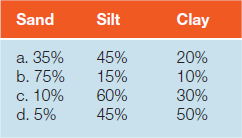Chapter 9, Problem 2PA

Chapter
Section
Textbook Problem

Refer to Figure 9.24. Using the texture triangle, determine the textures of the following soil samples.To determine

The textures of the given soil samples by referring Figure 9.24 from the textbook.

Explanation

Figure 9.24 shows the triangular graph that is used to differentiate different classes of soil texture based on the percentage of the sand, silt and clay within each class. The three sides of the triangle are plotted with percentages of sand, silt and clay from 0 to 100.

According to the graph, the soil sample-a, which has 35% of sand, 45% of silt and 20% of clay can be plotted under loam...

Still sussing out bartleby?

Check out a sample textbook solution.

See a sample solution

The Solution to Your Study Problems

Bartleby provides explanations to thousands of textbook problems written by our experts, many with advanced degrees!

Get Started

Find more solutions based on key concepts